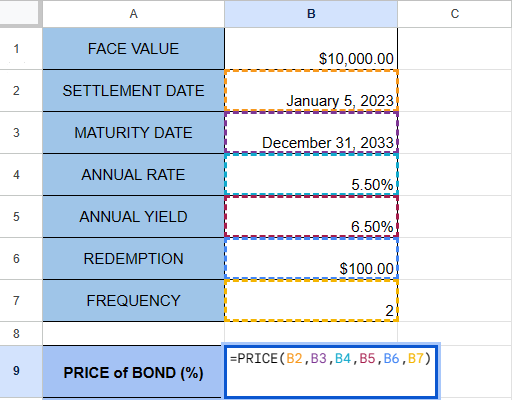# How to Use the PRICE Function in Google Sheets

This guide will explain how to use the PRICE function in Google Sheets.

Securities such as US Treasury bonds are popular investments because of their low risk and periodic interest payments to the investor in the form of coupon payments.

You can use the built-in `PRICE` function in Google Sheets to calculate the price of the security before purchasing it. The output of the `PRICE` function is the price per \$100 face value of a security that pays periodic interest.

In this guide, we will provide a step-by-step tutorial on how to use the `PRICE` function to determine the value of a security that pays periodic interest. We will also cover a few concrete examples of the function in use.

## The Anatomy of the PRICE Function

The syntax of the `PRICE` function is as follows:

`=PRICE(settlement, maturity, rate, yield, redemption, frequency, [day_count_convention])`

Let’s look at each argument to understand how to use the `PRICE` function.

• settlement refers to the settlement date of the given security. This is the date when the security is delivered to the buyer.
• The maturity parameter is the end date of the security.
• rate refers to the annualized rate of interest of the security.
• yield is the expected annual yield of the security
• redemption refers to the redemption value of the security.
• frequency refers to the number of interest payments made per year.
• day_count_convention is an optional argument allowing you to choose the day-count method to use.
• A value of 0 indicates the US (NASD) 30/360 standard that assumes months are 30 days long and a year is exactly 360 days.
• A value of 1 indicates the Actual/Actual standard where the actual number of days is used
• A value of 2 indicates the Actual/360 method which calculates using the actual number of days but assumes a 360-day year
• A value of 3 indicates the Actual/365 method which calculates using the actual number of days and assumes a 365-day year.
• A value of 4 indicates the European 30/360 method which calculates dates using a 30-day month and a 360-day year with adjustments for European financial conventions.

## A Real Example of Using the PRICE Function in Google Sheets

Let’s explore a simple example where we can use the `PRICE` function to calculate the price of a security paying periodic interest.

Suppose you have a bond with the following details:

• a settlement date of January 5th, 2023
• a maturity date of December 31, 2033
• an annual rate of 5.5%
• an annual yield of 6.5%
• and a redemption value of \$100

Given that the bond has a face value of \$10,000 and pays semi-annual payments to the investor, how much is the bond’s price?In the table above, we’ve created a table that lists all the data we need to compute for the price of the bond. Since semi-annual payments are paid twice a year, we’ll input a value of 2 for the frequency.We’ll input these details as arguments to our `PRICE` function to determine the price of our bond. We can use the following formula:

`=PRICE(B1,B2,B3,B4,B5,B6)`

The function reveals that our bond is priced at 92.23% of the face value. Since the optional day_count_convention field is not filled, our `PRICE` function will use the 30/360 method by default.To determine the actual dollar value of the bond, we can use the following formula:

`=B9/100 * B1`

The formula above divides the result of the `PRICE` function by 100 to create a percentage value. We’ll multiply this percentage by the face value of our bond to find the actual dollar value.

Click on the link below to create your own copy of our examples.

Head to the next section to read our step-by-step tutorial on how to create a weighted sales pipeline.

## How to Use the PRICE Function in Google Sheets

1. Create a table that lists the required information you need to calculate the price of the security. The settlement date and maturity date must be valid dates. The annual rate and annual yield must be a percentage and the frequency value must be either 1,2, or 4.To ensure that the dates are valid, we recommend using the `DATE` function to input the settlement and maturity dates.2. Type the `PRICE` function and enter the arguments starting from the settlement date up to the frequency value. In this example, we’ll use the formula =PRICE(B2,B3,B4,B5,B6,B7).3. Hit the Enter key to evaluate the `PRICE` function.4. To get the dollar value of the bond, divide the result of the `PRICE` function by 100 and multiply by the bond’s face value.These are all the steps you need to use the `PRICE` function to determine the value of a bond with periodic payments.

That’s all for this guide! Check out our library for more spreadsheet resources, tips, and tricks!Our goal this year is to create lots of rich, bite-sized tutorials for Excel users like you. If you liked this one, you'd love what we are working on! Readers receive early access to new content.

##### You May Also Like## How to Insert a Spin Button in Google Sheets

A spin button in a worksheet lets you quickly change and select new values for a cell. You…## How to Make Pixel Art and Emoji Art in Google Sheets

This guide will show you how you can use an add-on to make your very own pixel art…## How to Use POISSON.DIST Function in Google Sheets

The POISSON.DIST function in Google Sheets is useful when you want to return the value of the Poisson…## How To Remove Duplicates in Google Sheets

In this article, we’ll see how to remove duplicates in Google Sheets. Consider you have a massive amount…